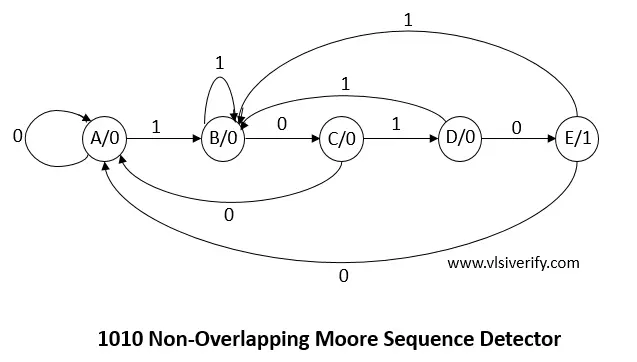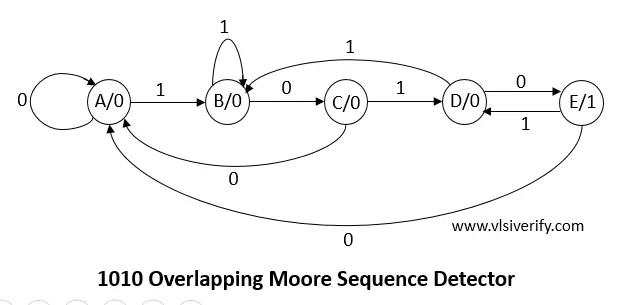Tutorials

In moore machine, output only depends on the present state. It is independent of current input. It is important to understand basics of finite state machine (FSM) and sequence detector.

## 1010 non-Overlapping Moore Sequence Detector Verilog Code``````module seq_detector_1010(input bit clk, rst_n, x, output z);
parameter A = 4'h1;
parameter B = 4'h2;
parameter C = 4'h3;
parameter D = 4'h4;
parameter E = 4'h5; // extra state when compared with Mealy Machine

bit [3:0] state, next_state;
always @(posedge clk or negedge rst_n) begin
if(!rst_n) begin
state <= A;
end
else state <= next_state;
end

always @(state or x) begin
case(state)
A: begin
if(x == 0) next_state = A;
else       next_state = B;
end
B: begin
if(x == 0) next_state = C;
else       next_state = B;
end
C: begin
if(x == 0) next_state = A;
else       next_state = D;
end
D: begin
if(x == 0) next_state = E;
else       next_state = B;
end
E: begin
if(x == 0) next_state = A;
else       next_state = B; //This state only differs when compared with Moore Overlaping Machine
end
default: next_state = A;
endcase
end
assign z = (state == D)? 1:0;
endmodule``````

## Testbench Code

``````module TB;
reg clk, rst_n, x;
wire z;

seq_detector_1010 sd(clk, rst_n, x, z);
initial clk = 0;
always #2 clk = ~clk;

initial begin
\$monitor("%0t: x = %0b, z = %0b", \$time, x, z);
x = 0;
#1 rst_n = 0;
#2 rst_n = 1;

#3 x = 1;
#4 x = 1;
#4 x = 0;
#4 x = 1;
#4 x = 0;
#4 x = 1;
#4 x = 0;
#4 x = 1;
#4 x = 0;
#4 x = 1;
#4 x = 0;
#10;
\$finish;
end

initial begin
// Dump waves
\$dumpfile("dump.vcd");
\$dumpvars(0);
end
endmodule``````

Output:

``````0: x = 0, z = 0
6: x = 1, z = 0
14: x = 0, z = 0
18: x = 1, z = 0
22: x = 0, z = 1
26: x = 1, z = 0
30: x = 0, z = 0
34: x = 1, z = 0
38: x = 0, z = 1
42: x = 1, z = 0
46: x = 0, z = 0``````

## 1010 Overlapping Moore Sequence Detector Verilog Code``````module seq_detector_1010(input bit clk, rst_n, x, output z);
parameter A = 4'h1;
parameter B = 4'h2;
parameter C = 4'h3;
parameter D = 4'h4;
parameter E = 4'h5; // extra state when compared with Mealy Machine

bit [3:0] state, next_state;
always @(posedge clk or negedge rst_n) begin
if(!rst_n) begin
state <= A;
end
else state <= next_state;
end

always @(state or x) begin
case(state)
A: begin
if(x == 0) next_state = A;
else       next_state = B;
end
B: begin
if(x == 0) next_state = C;
else       next_state = B;
end
C: begin
if(x == 0) next_state = A;
else       next_state = D;
end
D: begin
if(x == 0) next_state = E;
else       next_state = B;
end
E: begin
if(x == 0) next_state = A;
else       next_state = D;
end
default: next_state = A;
endcase
end
assign z = (state == D)? 1:0;
endmodule``````

## Testbench Code

``````module TB;
reg clk, rst_n, x;
wire z;

seq_detector_1010 sd(clk, rst_n, x, z);
initial clk = 0;
always #2 clk = ~clk;

initial begin
\$monitor("%0t: x = %0b, z = %0b", \$time, x, z);
x = 0;
#1 rst_n = 0;
#2 rst_n = 1;

#3 x = 1;
#4 x = 1;
#4 x = 0;
#4 x = 1;
#4 x = 0;
#4 x = 1;
#4 x = 0;
#4 x = 1;
#4 x = 0;
#4 x = 1;
#4 x = 0;
#10;
\$finish;
end

initial begin
// Dump waves
\$dumpfile("dump.vcd");
\$dumpvars(0);
end
endmodule``````

Output:

``````0: x = 0, z = 0
6: x = 1, z = 0
14: x = 0, z = 0
18: x = 1, z = 0
22: x = 0, z = 1
26: x = 1, z = 0
30: x = 0, z = 1
34: x = 1, z = 0
38: x = 0, z = 1
42: x = 1, z = 0
46: x = 0, z = 1
50: x = 0, z = 0``````

Verilog Codes